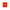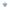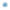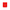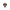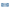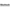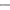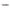# Geometric representation theory

## June 27—July 2, 2022

### Description

Location: HSE University Faculty of Mathematics, Skoltech Center for Advanced Studies.

Representation theory plays a key role in modern mathematics due to the huge number of applications in various fields of mathematics and mathematical physics, among which are integrable systems, quantum field theory, algebraic and convex geometry, and topology. The main idea of representation theory is to study various algebraic structures via their realization as symmetries of mathematical or physical objects. The opposite direction can also be used to derive algebraic, geometric, and combinatorial properties of an object via its symmetries. Geometric representation theory studies deep geometric properties of varieties, sheaves, and moduli spaces via the algebraic and combinatorial properties of algebraic groups, Lie algebras, quantum groups, or quivers. A classical example is the Borel-Weil-Bott theorem, which uniformly describes representations of simple Lie algebras via the line bundles on flag varieties.

### OBJECTIVES

We plan to bring together leading experts working in various fields of modern geometric representation theory, early career mathematicians, and graduate students to cover geometry and topology of the Grassmannians and flag varieties, representation theory of Kac-Moody Lie algebras, quiver varieties, representations of quivers, quantum groups, modular representation theory, applications in mathematical physics, and combinatorics.